### Home > CAAC > Chapter 3 > Lesson 3.1.3 > Problem3-24

3-24.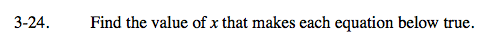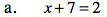Subtract 7 from both sides.

x = −5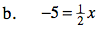Divide both sides by one half (otherwise known as multiplying by 2).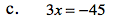Divide both sides by 3.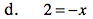Divide both sides by −1.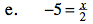Multiply both sides by 2.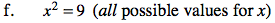Find the square root of both sides. Remember to include both the positive and negative values as you are taking the square root of a variable.